#### FeynRule

##### Description

FeynRule[lag, {fields}] derives the Feynman rule corresponding to the field configuration fields of the lagrangian lag.

``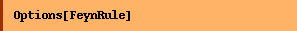``
`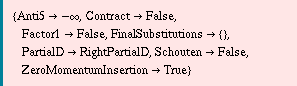`

FeynRule does not calculate propagator Feynman rules.

The option ZeroMomentumInsertion can be used for twist-2 and higher twist operators.

##### Examples
``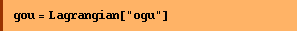``
`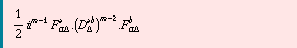`
``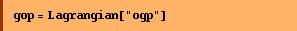``
`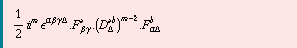`
``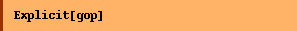``
`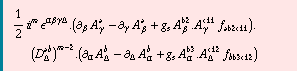`
``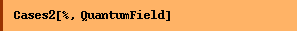``
`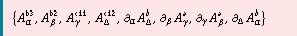`

2-gluon Feynman rules (unpolarized)

``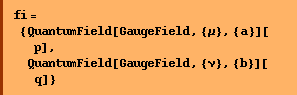``
`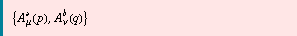`
``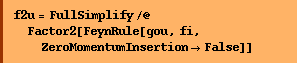``
`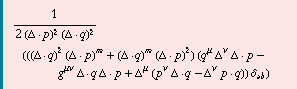`

2-gluon Feynman rules (polarized)

````
``
``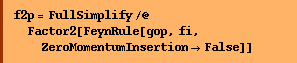``
`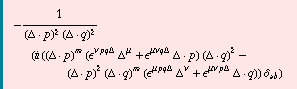`
``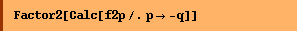``
`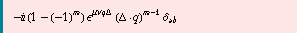`

Compare with the Feynman rule tabulated in Twist2GluonOperator.

``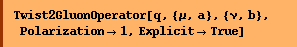``
`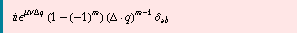`

quark-quark Feynman rule (unpolarized)

``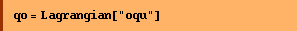``
`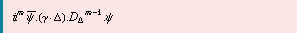`

quark-quark -gluon-gluon Feynman rule (unpolarized)

````
``
``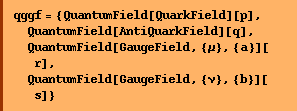``
`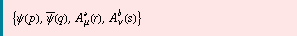`
``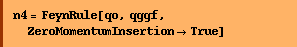``
`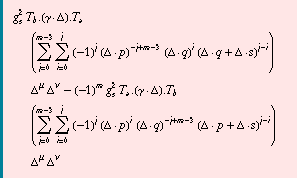`
``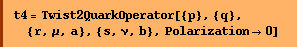``
`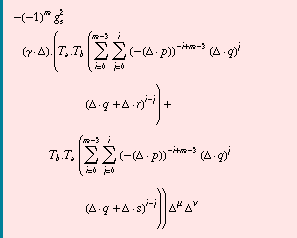`
``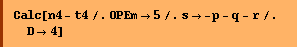``
`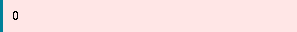`

In general equality can be shown by Timing[Factor2[ FCE[Calc[ChangeDimension[FCE[OPESumExplicit[n4-t4]],4]/.s->-p-q-r]]]] but it is a little bit slow ...

``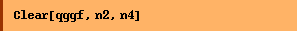``

QCD vertices

``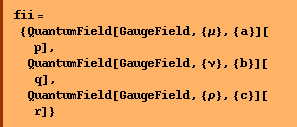``
`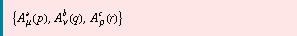`
``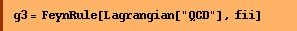``
`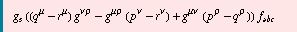`
``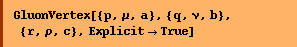``
`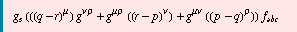`
``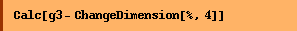``
``
``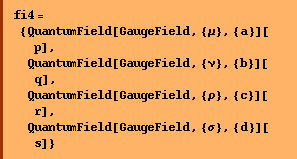``
`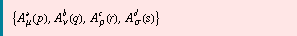`
``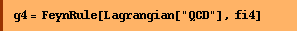``
``
``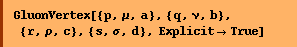``
``
``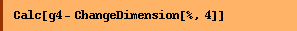``
``
``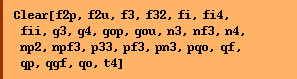``

Converted from the Mathematica notebook FeynRule.nb